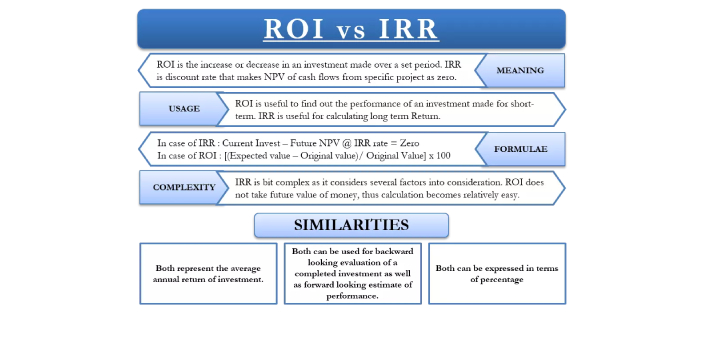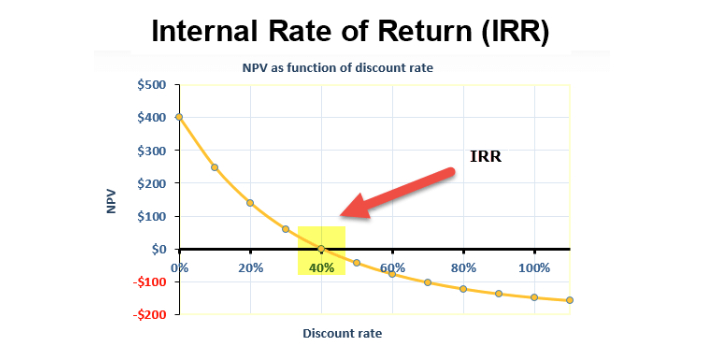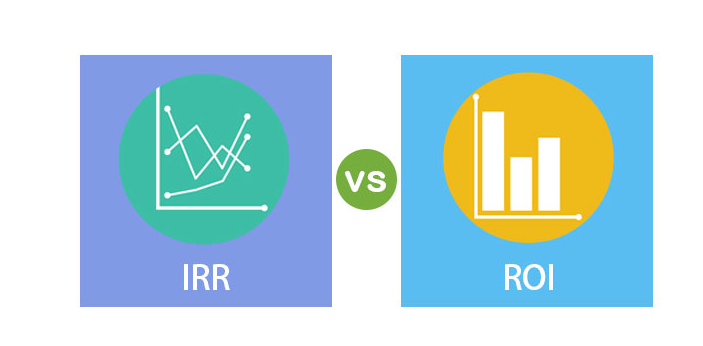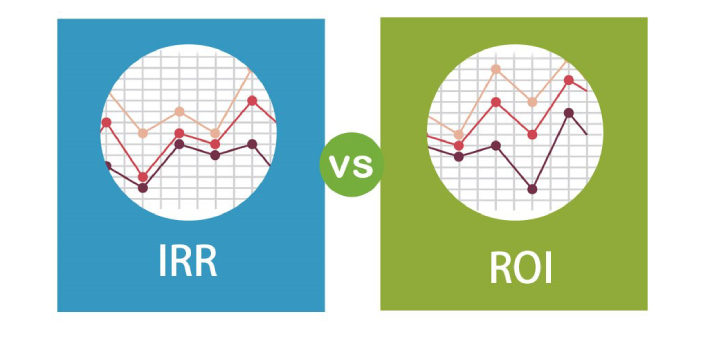What is the difference between IRR and ROI? - ProProfs Discuss+ Ask Question

# What is the difference between IRR and ROI?

Asked by R. Barnes, Last updated: Sep 15, 2023

###Request 0FollowShareAnswer AnonymouslyAnswer LaterCopy LinkN. Reyes, Writer, B.ed, CaliforniaIRR and ROI are known to be two terms that are used for investments. Those who are fond of investing may be familiar with these terms. When you say IRR, this means Internal Rate of Return, while when you say ROI, this means Return of Investment. IRR will provide you with the time value of money, which means that you will know what the growth rate of your investment is every year.With the ROI, you will not know how well your investments are doing until such time when you would already get it. There are different ways that you can compute for these two metrics, but there are also some calculators that are available online that will help you learn more about the investments that you are taking.H. Jones, Web Content Writer, San Antonio,

IRR is the acronym for Return of Investment and ROR stands for Rate of Return. These two terms do not mean the same thing. IRR is a complex finance metric for investment; it is used to calculate the rate of return on investment. IRR is much more accurate than ROI. IRR is calculated as the discount rate that makes the difference between current investment and future NPV zero. The major disadvantage of IRR is that it requires more work to calculate compared to ROI. ROI is finance metric for investment.ROI is often used to calculate the rate of performance of an investment over a specific period of time. The formula used for calculating ROI is ROI = {(Expected value – Original value)/Original value} * 100. A major disadvantage of ROI is that it does not take into account the future value of money in its calculations. It only makes use of two values and two operations.J. Pollock, Science Professor, Los Angeles

IRR means Internal Rate of Return while ROI means Return of Investment. IRR usually tries to compute the amount that you will get based on the discount that you will get. There is no constant formula that you can use to solve it and there are different factors that you need to consider for this. ROI will try to compute the potential investment rate that you will get over a certain period of time.In order to get the ROI, you need to follow this formula: ROI = [(Expected Value – Original Value) / Original Value] x 100. Things will be a lot less complicated as long as you would remember this formula when you are trying to compute certain figures.Bobby Rickets, Content Reviewer

IRR stands for Internal Rate of Return while ROI stands for Return of Investment. The ROI will show the total growth of what is being measured from the time that it started until it finishes. The IRR can be used to show the growth rate annually, which means that people make use of this every year.The numbers are supposed to be the same throughout the year, but there will be more differences as more years pass. There is usually a type of formula that is being followed when trying to compute the ROI, but for the IRR, a lot of people would need to do trial and error before they get the answers that they want.Search for Google imagesSelect a recommended image
Upload from your computerCancelSearch for Google imagesSelect a recommended image
Upload from your computerCancelSearch for Google imagesSelect a recommended image
Upload from your computerCancel